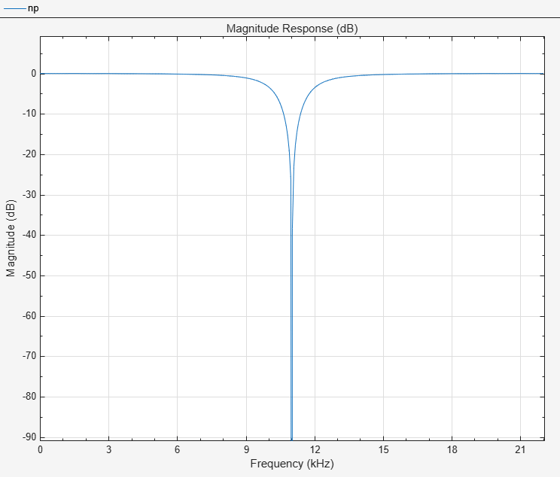# getQualityFactor

Get quality factor

## Syntax

``Q = getQualityFactor(npFilter)``

## Description

example

````Q = getQualityFactor(npFilter)` returns the quality factor (Q factor) of the notch peak filter. The Q factor is defined as the center frequency divided by the bandwidth.```

## Examples

collapse all

Create a `dsp.NotchPeakFilter` object in the default configuration, where the `Specification `property is set to `'Bandwidth and center frequency'`.

`np = dsp.NotchPeakFilter`
```np = dsp.NotchPeakFilter with properties: Specification: 'Bandwidth and center frequency' Bandwidth: 2205 CenterFrequency: 11025 SampleRate: 44100 ```

Determine the quality factor of the filter using the `getQualityFactor` function. The quality factor is given by the ratio of the center frequency to the bandwidth.

`getQualityFactor(np)`
```ans = 5 ```

Visualize the filter response using `fvtool`.

`fvtool(np)`## Input Arguments

collapse all

Notch peak filter whose quality factor is computed, specified as a `dsp.NotchPeakFilter` object.

## Output Arguments

collapse all

Quality factor of the filter, returned as a scalar. The Q factor is defined as the center frequency divided by the bandwidth.

Data Types: `double`

## Version History

Introduced in R2014a